# Posts tagged as “union find”

There are n computers numbered from 0 to n-1 connected by ethernet cables connections forming a network where connections[i] = [a, b] represents a connection between computers a and b. Any computer can reach any other computer directly or indirectly through the network.

Given an initial computer network connections. You can extract certain cables between two directly connected computers, and place them between any pair of disconnected computers to make them directly connected. Return the minimum number of times you need to do this in order to make all the computers connected. If it’s not possible, return -1.

Example 1:

Input: n = 4, connections = [[0,1],[0,2],[1,2]]
Output: 1
Explanation: Remove cable between computer 1 and 2 and place between computers 1 and 3.


Example 2:

Input: n = 6, connections = [[0,1],[0,2],[0,3],[1,2],[1,3]]
Output: 2


Example 3:

Input: n = 6, connections = [[0,1],[0,2],[0,3],[1,2]]
Output: -1
Explanation: There are not enough cables.


Example 4:

Input: n = 5, connections = [[0,1],[0,2],[3,4],[2,3]]
Output: 0


Constraints:

• 1 <= n <= 10^5
• 1 <= connections.length <= min(n*(n-1)/2, 10^5)
• connections[i].length == 2
• 0 <= connections[i], connections[i] < n
• connections[i] != connections[i]
• There are no repeated connections.
• No two computers are connected by more than one cable.

## Solution 1: Union-Find

Time complexity: O(V+E)
Space complexity: O(V)

## Solution 2: DFS

Time complexity: O(V+E)
Space complexity: O(V+E)

## C++

You are given a string s, and an array of pairs of indices in the string pairs where pairs[i] = [a, b] indicates 2 indices(0-indexed) of the string.

You can swap the characters at any pair of indices in the given pairs any number of times.

Return the lexicographically smallest string that s can be changed to after using the swaps.

Example 1:

Input: s = "dcab", pairs = [[0,3],[1,2]]
Output: "bacd"
Explaination:
Swap s and s, s = "bcad"
Swap s and s, s = "bacd"


Example 2:

Input: s = "dcab", pairs = [[0,3],[1,2],[0,2]]
Output: "abcd"
Explaination:
Swap s and s, s = "bcad"
Swap s and s, s = "acbd"
Swap s and s, s = "abcd"

Example 3:

Input: s = "cba", pairs = [[0,1],[1,2]]
Output: "abc"
Explaination:
Swap s and s, s = "bca"
Swap s and s, s = "bac"
Swap s and s, s = "abc"



Constraints:

• 1 <= s.length <= 10^5
• 0 <= pairs.length <= 10^5
• 0 <= pairs[i], pairs[i] < s.length
• s only contains lower case English letters.

## Solution: Connected Components

Use DFS / Union-Find to find all the connected components of swapable indices. For each connected components (index group), extract the subsequence of corresponding chars as a string, sort it and put it back to the original string in the same location.

e.g. s = “dcab”, pairs = [[0,3],[1,2]]
There are two connected components: {0,3}, {1,2}
subsequences:
1. 0,3 “db”, sorted: “bd”
2. 1,2 “ca”, sorted: “ac”
0 => b
1 => a
2 => c
3 => d
final = “bacd”

Time complexity: DFS: O(nlogn + k*(V+E)), Union-Find: O(nlogn + V+E)
Space complexity: O(n)

## C++/Union-Find

Given an array equations of strings that represent relationships between variables, each string equations[i] has length 4 and takes one of two different forms: "a==b" or "a!=b".  Here, a and b are lowercase letters (not necessarily different) that represent one-letter variable names.

Return true if and only if it is possible to assign integers to variable names so as to satisfy all the given equations.

Example 1:

Input: ["a==b","b!=a"]
Output: false
Explanation: If we assign say, a = 1 and b = 1, then the first equation is satisfied, but not the second.  There is no way to assign the variables to satisfy both equations.


Example 2:

Input: ["b==a","a==b"]
Output: true
Explanation: We could assign a = 1 and b = 1 to satisfy both equations.


Example 3:

Input: ["a==b","b==c","a==c"]
Output: true


Example 4:

Input: ["a==b","b!=c","c==a"]
Output: false


Example 5:

Input: ["c==c","b==d","x!=z"]
Output: true


Note:

1. 1 <= equations.length <= 500
2. equations[i].length == 4
3. equations[i] and equations[i] are lowercase letters
4. equations[i] is either '=' or '!'
5. equations[i] is '='

## Solution: Union Find

Time complexity: O(n)
Space complexity: O(1)

## C++

In a N x N grid composed of 1 x 1 squares, each 1 x 1 square consists of a /\, or blank space.  These characters divide the square into contiguous regions.

(Note that backslash characters are escaped, so a \ is represented as "\\".)

Return the number of regions.

Example 1:

Input:[  " /",  "/ "]Output: 2Explanation: The 2x2 grid is as follows:Example 2:

Input:[  " /",  "  "]Output: 1Explanation: The 2x2 grid is as follows: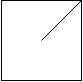Example 3:

Input:[  "\/",  "/\"]Output: 4Explanation: (Recall that because \ characters are escaped, "\/" refers to \/, and "/\" refers to /\.)The 2x2 grid is as follows: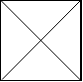Example 4:

Input:[  "/\",  "\/"]Output: 5Explanation: (Recall that because \ characters are escaped, "/\" refers to /\, and "\/" refers to \/.)The 2x2 grid is as follows: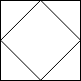Example 5:

Input:[  "//",  "/ "]Output: 3Explanation: The 2x2 grid is as follows: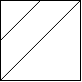Note:

1. 1 <= grid.length == grid.length <= 30
2. grid[i][j] is either '/''\', or ' '.

# Solution 1: Split grid into 4 triangles and Union Find Faces

Divide each grid into 4 triangles and union them if not split.
Time complexity: O(n^2*alphn(n^2))
Space complexity: O(n^2)

# Solution 3: Pixelation (Upscale 3 times)

Time complexity: O(n^2)
Space complexity: O(n^2)

# Problem

Given a non-empty array of unique positive integers A, consider the following graph:

• There are A.length nodes, labelled A to A[A.length - 1];
• There is an edge between A[i] and A[j] if and only if A[i] and A[j] share a common factor greater than 1.

Return the size of the largest connected component in the graph.

Example 1:

Input: [4,6,15,35]
Output: 4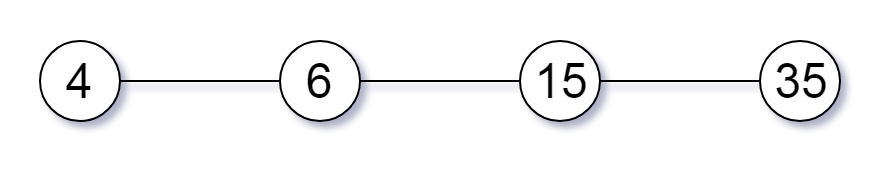Example 2:

Input: [20,50,9,63]
Output: 2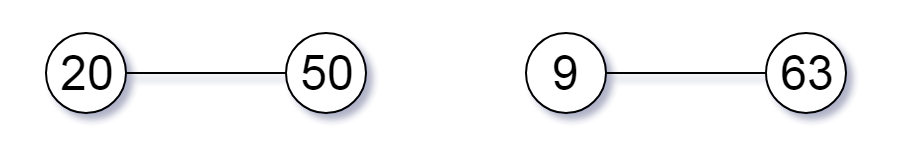Example 3:

Input: [2,3,6,7,4,12,21,39]
Output: 8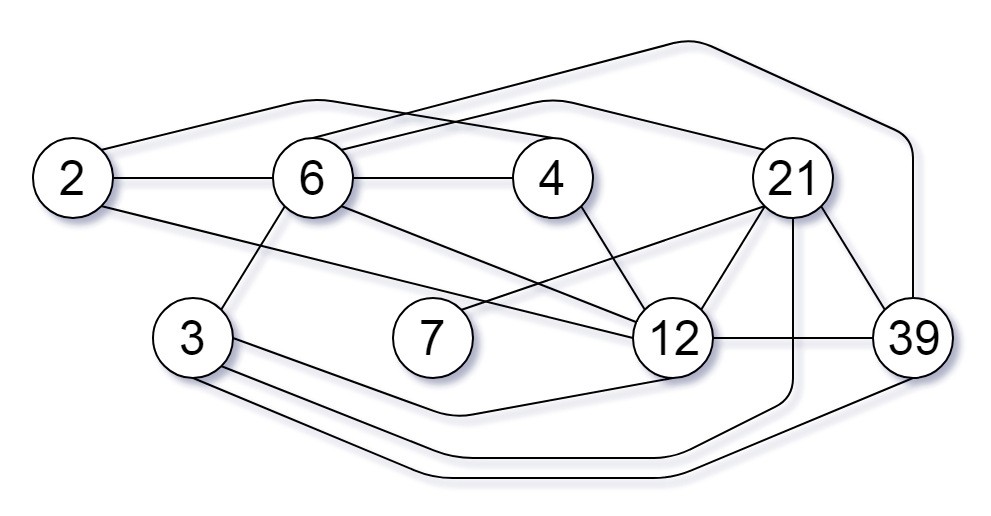Note:

1. 1 <= A.length <= 20000
2. 1 <= A[i] <= 100000

# Solution: Union Find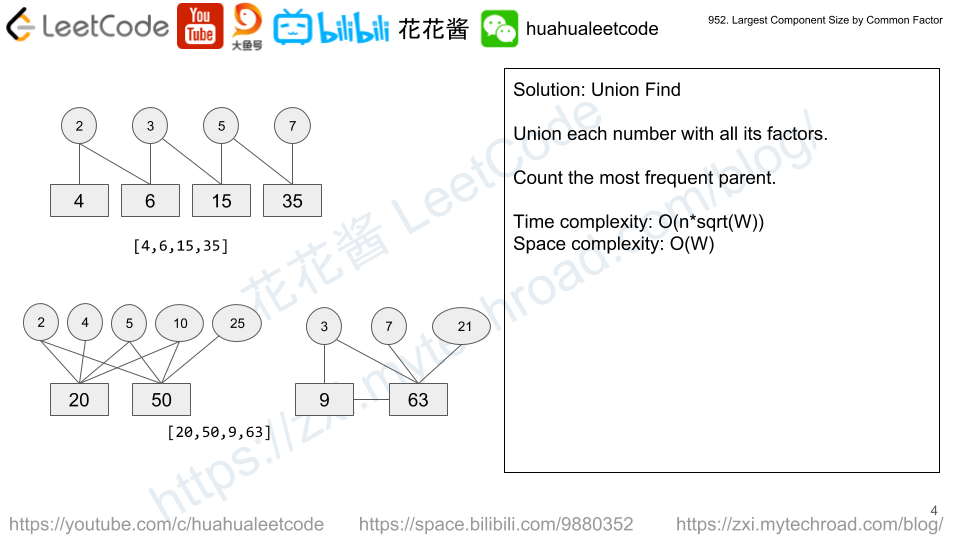For each number, union itself with all its factors.

E.g. 6, union(6,2), union(6,3)

Time complexity: $$O(\Sigma{sqrt(A[i])})$$

Space complexity: $$O(max(A))$$

## Python3

Mission News Theme by Compete Themes.